# HELP needed with Autocad lisp!!!

## Recommended PostsI am in a hurry with the project so I don't have time to write this lisp myself specially couse I don't have any experience in it, so if anyone can help that would be great. Anyway I have a lot of parcels drawn in autocad and what i need to do is trim all the polyline endings and intersections and insert a point in the middle. Manually I do it this way:

- first i make circles (radius has to be 0.5) on the endings and intersections of polylines

- then I trim all the lines inside the circles

- then I insert a point in the center of circles and at the end delete circles.

There is also a picture at the bottom showing the first and the last fase of the process.

If anyone knows about the lisp that does that or maybe have time to write one it would help me a lot.

##### Share on other sites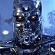Would it be possible to get the same affect if you created a block of a point and used a mask to block the ends of the lines? This block could then be inserted at each intersection.

In the future, to avoid a panic, it is best to seek an answer to a problem before you are on the threshold of your deadline. Just some friendly advice. No offense meant.

##### Share on other sitesCreate a block containing a wipeout and a point, insert it on all the corners and then turn off the wipeout frames.

Here is what it looks like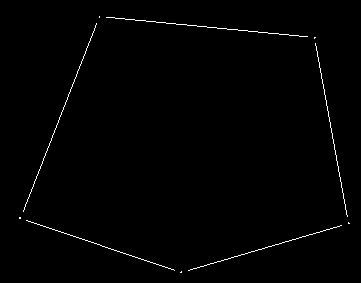##### Share on other sitesWhat he ^ ^ ^ said works for me. Plus he said it much better than I did too. LoL

##### Share on other sitesThanks but how do I make such a block? And can I insert it automaticly on every intersection and ending of polyline?

##### Share on other sitesYou make it like any other block??

Automatically insert the block at every intersection? Is that practical? How many intersections are there?

##### Share on other sitesSorry for a delay on answer. Didn't have internet. There are many intersections and I have to do the sam thing on all polyline endings so the number is big. That's why I asked is there a way to insert them automatically to save time. Anyways I know how to make a block but I don't understand how to make a block containing a whipeout?!

##### Share on other sitesIf you put in your thread title "Willing to Pay" you might get a better response.Or be patient... people are definitely generous but hope you can get someone to do something for you in time.

##### Share on other sitesGood idea but I think I'm gonna wait a little more! :-)

If you put in your thread title "Willing to Pay" you might get a better response.Or be patient... people are definitely generous but hope you can get someone to do something for you in time.

##### Share on other sitesHere is some hastily written code, but should perform as required: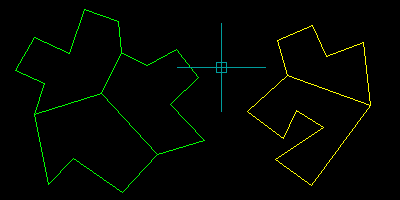```([color=BLUE]defun[/color] c:trimparcel ( [color=BLUE]/[/color] a c d e h i l p s v )
([color=BLUE]if[/color] ([color=BLUE]setq[/color] s ([color=BLUE]ssget[/color] [color=MAROON]"_:L"[/color] '((0 . [color=MAROON]"LWPOLYLINE"[/color]))))
([color=BLUE]repeat[/color] ([color=BLUE]setq[/color] i ([color=BLUE]sslength[/color] s))
([color=BLUE]setq[/color] e ([color=BLUE]ssname[/color] s ([color=BLUE]setq[/color] i ([color=BLUE]1-[/color] i)))
d ([color=BLUE]entget[/color] e)
h ([color=BLUE]list[/color]
([color=BLUE]assoc[/color] 8 d)
([color=BLUE]cond[/color] (([color=BLUE]assoc[/color] 06 d)) ('(06 . [color=MAROON]"BYLAYER"[/color])))
([color=BLUE]cond[/color] (([color=BLUE]assoc[/color] 62 d)) ('(62 . 256)))
)
v [color=BLUE]nil[/color]
)
([color=BLUE]while[/color] ([color=BLUE]setq[/color] a ([color=BLUE]assoc[/color] 10 d))
([color=BLUE]setq[/color] p ([color=BLUE]cdr[/color] a)
v ([color=BLUE]cons[/color] p v)
d ([color=BLUE]cdr[/color] ([color=BLUE]member[/color] a d))
)
([color=BLUE]if[/color] ([color=BLUE]not[/color] ([color=BLUE]vl-some[/color] '([color=BLUE]lambda[/color] ( x ) ([color=BLUE]equal[/color] p x 1e-) l))
([color=BLUE]progn[/color]
([color=BLUE]entmake[/color] ([color=BLUE]vl-list*[/color] '(0 . [color=MAROON]"POINT"[/color]) ([color=BLUE]cons[/color] 10 p) h))
([color=BLUE]setq[/color] l ([color=BLUE]cons[/color] p l))
)
)
)
([color=BLUE]if[/color] ([color=BLUE]=[/color] 1 ([color=BLUE]logand[/color] 1 ([color=BLUE]cdr[/color] ([color=BLUE]assoc[/color] 70 ([color=BLUE]entget[/color] e)))))
([color=BLUE]setq[/color] v ([color=BLUE]cons[/color] ([color=BLUE]last[/color] v) v))
)
([color=BLUE]mapcar[/color]
([color=BLUE]function[/color]
([color=BLUE]lambda[/color] ( a b [color=BLUE]/[/color] x )
([color=BLUE]if[/color]
([color=BLUE]not[/color]
([color=BLUE]vl-some[/color]
([color=BLUE]function[/color]
([color=BLUE]lambda[/color] ( x )
([color=BLUE]or[/color] ([color=BLUE]and[/color] ([color=BLUE]equal[/color] a ([color=BLUE]car[/color] x) 1e- ([color=BLUE]equal[/color] b ([color=BLUE]cadr[/color] x) 1e-)
([color=BLUE]and[/color] ([color=BLUE]equal[/color] b ([color=BLUE]car[/color] x) 1e- ([color=BLUE]equal[/color] a ([color=BLUE]cadr[/color] x) 1e-)
)
)
)
c
)
)
([color=BLUE]progn[/color]
([color=BLUE]setq[/color] c ([color=BLUE]cons[/color] ([color=BLUE]list[/color] a b) c)
x ([color=BLUE]angle[/color] a b)
)
([color=BLUE]entmake[/color]
([color=BLUE]vl-list*[/color]
'(0 . [color=MAROON]"LINE"[/color])
([color=BLUE]cons[/color] 10 ([color=BLUE]polar[/color] a x 0.5))
([color=BLUE]cons[/color] 11 ([color=BLUE]polar[/color] b ([color=BLUE]+[/color] x [color=BLUE]pi[/color]) 0.5))
h
)
)
)
)
)
)
v ([color=BLUE]cdr[/color] v)
)
([color=BLUE]entdel[/color] e)
)
)
([color=BLUE]princ[/color])
)
([color=BLUE]princ[/color])
```

Following the theme of Styk's post, perhaps make a contribution to my site if the above saves you time.

Edited by Lee Mac

##### Share on other sitesAnother ....```(defun c:Test (/ ang i in e j p p1 p2 p3 p4 pts sn ss)
;;; Tharwat 29. September. 2012   ;;;
(if (setq ss (ssget '((0 . "*POLYLINE"))))
(repeat (setq in (sslength ss))
(setq sn (ssname ss (setq in (1- in))))
(setq e (entget sn))
(repeat (setq i (fix (vlax-curve-getendparam sn)))
(setq pts (cons (vlax-curve-getpointatparam sn i) pts))
(setq i (1- i))
)
(setq j 0)
(repeat (1- (length pts))
(setq ang (angle (setq p1 (nth j pts))
(setq p2 (nth (setq j (1+ j)) pts))
)
)
(setq
p (cons (list (setq p3
(polar (mapcar '(lambda (a b) (/ (+ a b) 2.)) p1 p2)
ang
(- (/ (distance p1 p2) 2.) 0.5)
)
)
(polar p3 (+ ang pi) (- (distance p1 p2) 1.0))
)
p
)
)
)
(setq
p (cons (list (setq p4
(polar (mapcar '(lambda (a b) (/ (+ a b) 2.))
(car pts)
(last pts)
)
(angle (car pts) (last pts))
(- (/ (distance (car pts) (last pts)) 2.) 0.5)
)
)
(polar p4
(+ (angle (car pts) (last pts)) pi)
(- (distance (car pts) (last pts)) 1.0)
)
)
p
)
)
(foreach x p
(entmakex (list '(0 . "LINE")
(cons 10 (car x))
(cons 11 (cadr x))
(assoc 8 e)
)
)
)
(foreach prm pts
(entmakex (list '(0 . "POINT") (cons 10 prm) (assoc 8 e)))
)
(entdel sn)
(setq pts nil
p   nil
)
)
(princ)
)
(princ)
)

```

##### Share on other sitesHere is some hastily written code, but should perform as required:

Thank you very much Lee Mac, you are a genious. I'm not big on money at the moment but i will make a small contribution to you website. Thanks ones again! :-)

##### Share on other sitesThank you Tharwat for your time. An error occurs when I try to use your lisp "error: no function definition: VLAX-CURVE-GETENDPARAM". A you can se by my reply to lee mac his lisp works so you can compare and see where is the problem. Anyways thanks.

Another ....```(defun c:Test (/ ang i in e j p p1 p2 p3 p4 pts sn ss)
;;; Tharwat 29. September. 2012   ;;;
(if (setq ss (ssget '((0 . "*POLYLINE"))))
(repeat (setq in (sslength ss))
(setq sn (ssname ss (setq in (1- in))))
(setq e (entget sn))
(repeat (setq i (fix (vlax-curve-getendparam sn)))
(setq pts (cons (vlax-curve-getpointatparam sn i) pts))
(setq i (1- i))
)
(setq j 0)
(repeat (1- (length pts))
(setq ang (angle (setq p1 (nth j pts))
(setq p2 (nth (setq j (1+ j)) pts))
)
)
(setq
p (cons (list (setq p3
(polar (mapcar '(lambda (a b) (/ (+ a b) 2.)) p1 p2)
ang
(- (/ (distance p1 p2) 2.) 0.5)
)
)
(polar p3 (+ ang pi) (- (distance p1 p2) 1.0))
)
p
)
)
)
(setq
p (cons (list (setq p4
(polar (mapcar '(lambda (a b) (/ (+ a b) 2.))
(car pts)
(last pts)
)
(angle (car pts) (last pts))
(- (/ (distance (car pts) (last pts)) 2.) 0.5)
)
)
(polar p4
(+ (angle (car pts) (last pts)) pi)
(- (distance (car pts) (last pts)) 1.0)
)
)
p
)
)
(foreach x p
(entmakex (list '(0 . "LINE")
(cons 10 (car x))
(cons 11 (cadr x))
(assoc 8 e)
)
)
)
(foreach prm pts
(entmakex (list '(0 . "POINT") (cons 10 prm) (assoc 8 e)))
)
(entdel sn)
(setq pts nil
p   nil
)
)
(princ)
)
(princ)
)

```

##### Share on other sitesHere is some hastily written code, but should perform as required:

Thank you very much Lee Mac, you are a genious. I'm not big on money at the moment but i will make a small contribution to you website. Thanks ones again! :-)

Many thanks ruso!

I'm glad that my code performs as required and I appreciate your contribution!

##### Share on other sitesAn error occurs when I try to use your lisp "error: no function definition: VLAX-CURVE-GETENDPARAM".

Just add (vl-load-com) to the routine and try agian .

##### Share on other sitesJust add (vl-load-com) to the routine and try agian .

Where in the routine? At the beginning at the end... ? I guess i should know that but like I said no experience what so ever so be patient with me! :-)

##### Share on other sitesJust type (vl-load-com) before you execute routine, or add it as first or last line whatsoever if you load it as *.lsp - it will load and that line too as also the rest of the code - main routine body (defun c:functionname (... / ... ) )

M.R.

##### Share on other sitesAnother ....

```(defun c:Test (/ ang i in e j p p1 p2 p3 p4 pts sn ss)
;;; Tharwat 29. September. 2012   ;;;
...```

A few issues with your code Tharwat: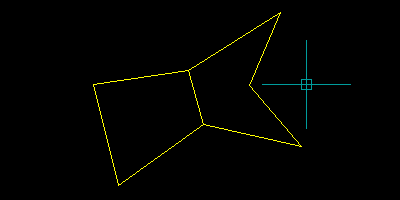##### Share on other sitesA few issues with your code Tharwat:

That's correct and I have to rework on the code once again .

Yours also make duplicate lines on the joined side .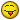##### Share on other sitesYours also make duplicate lines on the joined side

Good catch - I have now updated my earlier code.

## Join the conversation

You can post now and register later. If you have an account, sign in now to post with your account.
Note: Your post will require moderator approval before it will be visible.Reply to this topic...

×   Pasted as rich text.   Paste as plain text instead

Only 75 emoji are allowed.